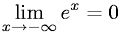Equations > Calculus > Limits > Limit of e to the X power as X Approaches Negative Infinity

### Limit of e to the X power as X Approaches Negative InfinityLatex Code:

MathML Code:

 $\underset{x\to -\infty }{lim}{e}^{x}=0$

MathType 5.0: